# Fractions

Students understand fractions as numbers on the number line and explore fraction equivalence and comparison in simple cases, then apply this fractional understanding to measure fractional lengths and represent them on a number line plot.

Math

Unit 6

## Unit Summary

In Unit 6, 3rd grade students extend and deepen 1st-grade work with understanding halves and fourths/quarters (1.G.3) as well as 2nd grade practice with equal shares of halves, thirds, and fourths (2.G.3) to understanding fractions as numbers. Their knowledge becomes more formal as they work with area models and the number line. Throughout the unit, students have multiple experiences working with the 3rd grade specified fractional units of halves, thirds, fourths, sixths, and eighths. To build flexible thinking about fractions, students are exposed to additional fractional units such as fifths, ninths, and tenths.

Students begin the unit by partitioning different models (like area models and fraction strips) of wholes into equal parts (3.G.2), allowing this supporting cluster content to enhance the major work of 3rd grade with fractions. They identify equal parts as halves, fourths, thirds, sixths, and eighths, then are introduced to fraction form and write unit fractions in the form $$\frac{1}{b}$$ (3.NF.1). Next, they make copies of unit fractions to build non-unit fractions, understanding unit fractions as the basic building blocks that compose other fractions (3.NF.1). Then, their focus shifts to fractions on the number line, a particularly important representation that helps students see fractions as numbers. They begin by using the interval from 0 to 1 as the whole and then extend to mark fractions beyond a whole. Noticing that some fractions with different units are placed at the exact same point on the number line, they come to understand equivalent fractions (3.NF.3a). Students express whole numbers as fractions and recognize fractions that are equivalent to whole numbers. Next, students use their understanding of the number of units and the size of each unit to compare fractions in simple cases, such as when dealing with common numerators or common denominators by reasoning about their size (3.NF.3d). Lastly, students measure lengths with fractional units and use data generated by measuring multiple objects to create line plots (3.MD.4). Lastly, students “use their developing knowledge of fractions and number lines to... [work] with measurement data involving fractional measurement values” (MD Progression, p. 10), and use that measurement data to create line plots (3.MD.4), thus using this supporting cluster work to enhance the major work of fractions.

This unit affords ample opportunity for students to engage with the Standards for Mathematical Practice. Students will develop an extensive toolbox of ways to model fractions, including area models, tape diagrams, and number lines (MP.5), choosing one model over another to represent a problem based on its inherent advantages and disadvantages. Students construct viable arguments and critique the reasoning of others as they explain why fractions are equivalent and justify their conclusions of a comparison with a visual fraction model (MP.3). They attend to precision as they come to more deeply understand what is meant by equal parts, and being sure to specify the whole when discussing equivalence and comparison (MP.6). Lastly, in the context of line plots, “measuring and recording data require attention to precision (MP.6)” (MD Progression, p. 3).

Unfortunately, “the topic of fractions is where students often give up trying to understand mathematics and instead resort to rules” (Van de Walle, p. 203). Thus, this unit places a strong emphasis on developing conceptual understanding of fractions, using the number line to represent fractions and to aid in students' understanding of fractions as numbers. With this strong foundation, students will operate on fractions in 4th grade and 5th grade (4.NF.3—4, 5.NF.1—7) and apply this understanding in a variety of contexts, such as proportional reasoning in middle school and interpreting functions in high school, among many others.

Pacing: 27 instructional days (24 lessons, 2 flex days, 1 assessment day)

Fishtank Plus for Math

Unlock features to optimize your prep time, plan engaging lessons, and monitor student progress.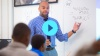## Assessment

The following assessments accompany Unit 6.

### Pre-Unit

Have students complete the Pre-Unit Assessment and Pre-Unit Student Self-Assessment before starting the unit. Use the Pre-Unit Assessment Analysis Guide to identify gaps in foundational understanding and map out a plan for learning acceleration throughout the unit.

### Mid-Unit

Have students complete the Mid-Unit Assessment after lesson 11.

### Post-Unit

Use the resources below to assess student understanding of the unit content and action plan for future units.

Expanded Assessment Package

Use student data to drive your planning with an expanded suite of unit assessments to help gauge students’ facility with foundational skills and concepts, as well as their progress with unit content.

## Unit Prep

### Intellectual Prep

Unit Launch

Prepare to teach this unit by immersing yourself in the standards, big ideas, and connections to prior and future content. Unit Launches include a series of short videos, targeted readings, and opportunities for action planning.#### Intellectual Prep for All Units

• Read and annotate “Unit Summary” and “Essential Understandings” portion of the unit plan.
• Do all the Target Tasks and annotate them with the “Unit Summary” and “Essential Understandings” in mind.
• Take the Post-Unit Assessment.

#### Unit-Specific Intellectual Prep

 Area model Example: The following shape represents 1 whole. $$\frac{1}{6}$$ of it is shaded.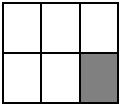Fraction strip/tape diagram Example: The following shape represents 1 whole. $$\frac{1}{6}$$ of it is shaded.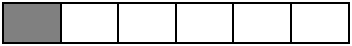Number line Example: The point on the number line below is located at $$\frac{1}{6}$$.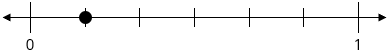Line plot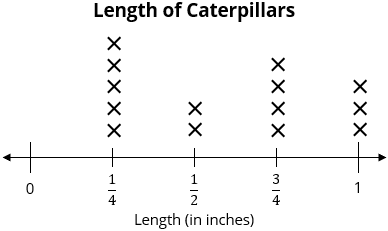### Essential Understandings

• “Unit fractions [are] basic building blocks for fractions, in the same sense that the number 1 is the basic building block of the whole numbers. Just as every whole number can be obtained by combining ones, every fraction can be obtained by combining copies of one unit fraction” (NF Progression, p. 7).
• Number line conventions that exist for whole numbers also apply to number lines that represent fractions. In other words, "just as 5 is the point on the number line reached by marking off 5 times the length of the unit interval from 0, so $$\frac{5}{3}$$ is the point obtained in the same way using a different interval as the unit of measurement, namely the interval from 0 to $$\frac{1}{3}$$” (NF Progression, p. 8).
• With both equivalence and comparison of fractions, it is important to make sure that each fraction refers to the same whole. For example, it is possible for a fourth of a large pizza to be greater than half of a small pizza.
• One can compare fractions with the same denominator by thinking about the number of units. For example, just as 5 inches is greater than 3 inches because it has a greater measurement in the same size, 5 eighths is greater than 3 eighths because it is made of more unit fractions of the same size.
• One can compare fractions with the same numerator by thinking about the size of the unit. For example, just as 2 inches is greater than 2 centimeters because inches are larger than centimeters, 2 thirds is greater than 2 fifths because thirds are larger than fifths.
• The numerical axis of a line plot is a segment of a number line. Further, “the number line diagram in a line plot corresponds to the scale on the measurement tool used to generate the data” (MD Progression, p 3).

### Vocabulary

denominator

equivalent fractions

fraction

numerator

unit fraction

To see all the vocabulary for Unit 6, view our 3rd Grade Vocabulary Glossary.

### Materials

• Optional: Template: Fair Shares (1 per student)
• Rectangular pieces of paper (6 per student) — These can be any size but must be the same size for all students. You could use a quarter piece of paper or an unlined index card for each.
• Strips of paper (5 per student) — These should measure about 5.5" by 1"
• Square-sized paper (1 per student)
• Optional: Square inch tiles (at least 4 per student) — You could also provide square inches cut out from Template: Square Inch Grid if you do not have enough square inch tiles, which should be cut into pieces before the lesson. See Lesson 5 for more information.
• Straightedge (1 per student) — This can be any tool used to draw a straight line, e.g., a straightedge, a ruler, etc.
• Fraction Cards (without pictures) (1 per pair of students)
• Fraction Cards (with pictures) (1 per pair of students)
• Template: Comparing Fractions Symbols (1 per pair of students)
• Template: Ruler (1 per every 6 students) —Students will construct quarter-inch rulers from these templates. You might choose to have quarter-inch rulers on hand for ease of using them to measure objects to the nearest quarter inch, but they are not required.

## Unit Practice

Word Problems and Fluency Activities

Access daily word problem practice and our content-aligned fluency activities created to help students strengthen their application and fluency skills.## Lesson Map

Topic A: Understanding Unit Fractions and Building Non-Unit Fractions

Topic B: Fractions on a Number Line

Topic C: Equivalent Fractions

Topic D: Comparing Fractions

Topic E: Line Plots

## Common Core Standards

Key

Major Cluster

Supporting Cluster

### Core Standards

#### Geometry

• 3.G.A.2 — Partition shapes into parts with equal areas. Express the area of each part as a unit fraction of the whole. For example, partition a shape into 4 parts with equal area, and describe the area of each part as 1/4 of the area of the shape.

#### Measurement and Data

• 3.MD.B.4 — Generate measurement data by measuring lengths using rulers marked with halves and fourths of an inch. Show the data by making a line plot, where the horizontal scale is marked off in appropriate units— whole numbers, halves, or quarters.

#### Number and Operations—Fractions

• 3.NF.A — Develop understanding of fractions as numbers.
• 3.NF.A.1 — Understand a fraction 1/b as the quantity formed by 1 part when a whole is partitioned into b equal parts; understand a fraction a/b as the quantity formed by a parts of size 1/b.
• 3.NF.A.2 — Understand a fraction as a number on the number line; represent fractions on a number line diagram.
• 3.NF.A.2.A — Represent a fraction 1/b on a number line diagram by defining the interval from 0 to 1 as the whole and partitioning it into b equal parts. Recognize that each part has size 1/b and that the endpoint of the part based at 0 locates the number 1/b on the number line.
• 3.NF.A.2.B — Represent a fraction a/b on a number line diagram by marking off a lengths 1/b from 0. Recognize that the resulting interval has size a/b and that its endpoint locates the number a/b on the number line.
• 3.NF.A.3 — Explain equivalence of fractions in special cases, and compare fractions by reasoning about their size.
• 3.NF.A.3.A — Understand two fractions as equivalent (equal) if they are the same size, or the same point on a number line.
• 3.NF.A.3.B — Recognize and generate simple equivalent fractions, e.g., 1/2 = 2/4, 4/6 = 2/3). Explain why the fractions are equivalent, e.g., by using a visual fraction model.
• 3.NF.A.3.C — Express whole numbers as fractions, and recognize fractions that are equivalent to whole numbers. Example: express 3 in the form 3 = 3/1; recognize that 6/1 = 6. Example: locate 4/4 and 1 at the same point of a number line diagram.
• 3.NF.A.3.D — Compare two fractions with the same numerator or the same denominator by reasoning about their size. Recognize that comparisons are valid only when the two fractions refer to the same whole. Record the results of comparisons with the symbols >, =, or <, and justify the conclusions, e.g., by using a visual fraction model.

• 2.G.A.3

• 2.MD.A.1
• 2.MD.A.2
• 2.MD.B.6
• 2.MD.D.9

• 4.MD.B.4

• 4.NF.A
• 4.NF.B

• 6.NS.C.6

### Standards for Mathematical Practice

• CCSS.MATH.PRACTICE.MP1 — Make sense of problems and persevere in solving them.

• CCSS.MATH.PRACTICE.MP2 — Reason abstractly and quantitatively.

• CCSS.MATH.PRACTICE.MP3 — Construct viable arguments and critique the reasoning of others.

• CCSS.MATH.PRACTICE.MP4 — Model with mathematics.

• CCSS.MATH.PRACTICE.MP5 — Use appropriate tools strategically.

• CCSS.MATH.PRACTICE.MP6 — Attend to precision.

• CCSS.MATH.PRACTICE.MP7 — Look for and make use of structure.

• CCSS.MATH.PRACTICE.MP8 — Look for and express regularity in repeated reasoning.

Unit 5

Shapes and Their Perimeter

Unit 7

Measurement

## Request a Demo

See all of the features of Fishtank in action and begin the conversation about adoption.

Yes

No

### Any other information you would like to provide about your school?